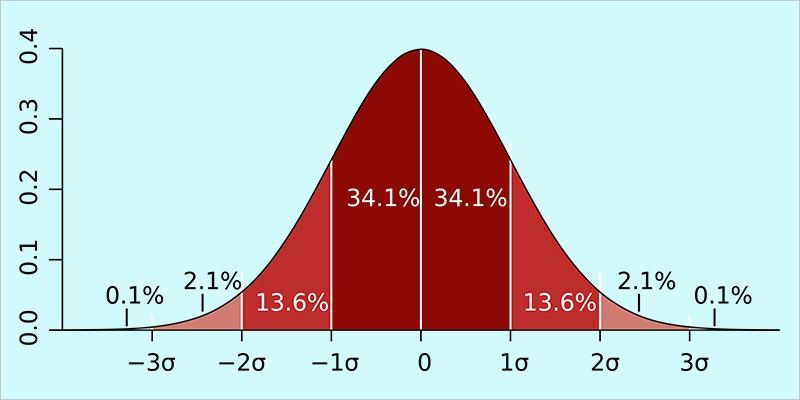# Measure of Dispersion in Statistics

### What is Measure of Dispersion?

The measure of central tendencies (like mean median and mode) generally describe the concentration of data set around the middle point. Although the central tendency is useful but to describe a set of data which is not complete. The information contained in a collection of data becomes more explanatory if the spread or the scatter of the set of data is also known.Similar to measures of central tendency, a particular value that describes the variability of a collection of data describes the measure of dispersion. The variability in data is measured using the values of the set of data along with the help of central tendency measures.

Generally, the parameters that can describe measures of variability are:

i) Range

ii) Quartiles

iii) Mean Deviation

We will study about all these one by one. Let us study about Range in the upcoming discussion.

Range:

Range gives us an approximate idea about the dispersion or scattering of data. To know what Range is, consider the following set of data:

The height (in cm.) of first 10 students (according to Roll Number) of three sections of class 12this given as follows:

 Height(in cm) Roll No. Class 12th (Section A) Class 12th(Section B) Class 12th(Section C) 1 160 156 166 2 143 155 169 3 154 143 165 4 122 160 143 5 147 147 141 6 176 158 145 7 166 153 143 8 160 149 156 9 163 170 155 10 139 156 164

Now, to find the range for different sections we need to calculate the difference between the maximum and the minimum value of different sections of the class.

So the range for any section of class 12th

Range for section A = 176 – 122 = 54 cm

Range for section B = 170 – 143 = 27 cm

Range for section C = 169 – 141 = 28 cm

Clearly, from the above example, Range for section A > Range for section C > Range for section B

Therefore, it can be said that the height of students is more dispersed or scattered in section A as compared to B or C.

Thus, we can define the range as the difference between the maximum and minimum values of a set of data. Although it gives us an approximate idea about the scattering of data but from the point of view of the central tendency, there are other methods to calculate a measure of variability. To know more about the measures of variability and Probability, stay in touch with byju’s. Everything you need is just a click away.